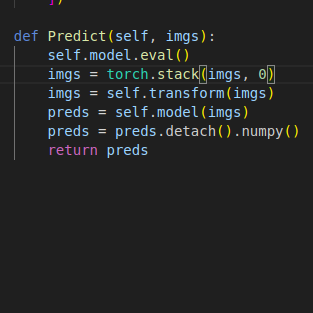# How to infernece pytorch model on list of images?

I have a list of numpy arrays each one represent an image and I want to predict all of them at same time.
Here’s my code:But I got this error:
imgs = torch.stack(imgs, 0)
TypeError: expected Tensor as element 0 in argument 0, but got numpy.ndarray

PyTorch doesnt work directly on numpy arrays, you need to convert them to torch tensors first, so as a first line you can add something like `imgs = [torch.from_numpy(img) for img in imgs]`

ok but the imgs is still a python list and can’t be fed to tranform or to the model itself

``````def predict(imgs, device):
model.eval()